# ISEE Upper Level Math : How to find the ratio of diameter and circumference

## Example Questions

### Example Question #1 : Diameter

A circle has a radius of. What is the ratio of the diameter to the circumference?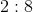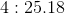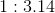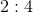Explanation:

The information about the radius is unnecessary to the problem. The equation of the circumference is: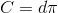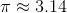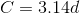Therefore, the circumference is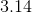times larger than the diameter, and the ratio of the diameter to the circumference is:### Example Question #2 : How To Find The Ratio Of Diameter And Circumference

A circle has a radius of. What is the ratio of the diameter to the circumference?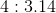Explanation:

The information about the radius is unnecessary to the problem. The equation of the circumference is:Therefore, the circumference istimes larger than the diameter, and the ratio of the diameter to the circumference is:### All ISEE Upper Level Math Resources